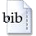User: GuestLogin
Original title:
The inclusive radiative B -> X_s gamma decay in the Standard Model
Translated title:
Der inklusive Zerfall B -> X_s gamma im Standard Modell
Author:
Haisch, UlrichYear:
2002
Document type:
Dissertation
Institution:
Fakultät für Physik
Buras, Andrzej Jerzy (Prof. Dr.)
Referee:
Buras, Andrzej Jerzy (Prof. Dr.); Drees, Manuel (Prof. Dr.)
Format:
Text
Language:
en
Subject group:
PHY Physik
Controlled terms:
B-Meson Zerfall; Schwache Wechselwirkung; Strange-Teilchen; Photon; Verzweigungsverhältnis; Berechnung; Standardmodell
Abstract:
We discuss inclusive radiative B decays within the Standard Model of elementary particle physics. In particular, we consider the B -> X_s gamma mode, where X_s denotes an arbitrary state of total strangeness -1. At quark level, this decay is dominated by the flavor changing neutral current b -> s gamma transition, which appears at the one-loop level in the Standard Model. Such processes allow us to study CP violation and the interplay of strong and electroweak interactions, to determine the elements of the quark mixing matrix, and to search for new physics. As it is well-known the inclusive rate is of much more theoretical interest than the exclusive modes, because non-perturbative effects play a subdominant role and are well under control due to heavy quark expansion. In particular, the decay width Gamma(B -> X_s gamma) is well approximated by the partonic decay rate Gamma(b -> s gamma) which can be analyzed in renormalization group improved perturbation theory. Exclusive decay modes, however, are more accessible to experiments, in particular at hadronic machines. We review the status quo of the theoretical prediction of the weak radiative B-meson decay B -> X_s gamma in the Standard Model. In particular, we point out that the charm contribution to B -> X_s gamma is numerically dominant and practically renormalization scale independent. The well-known enormous enhancement of the branching ratio by leading large logarithms is mainly due to the b-quark mass evolution in the top sector. By splitting the top and the charm contributions to the decay amplitude good control of the behavior of the perturbation series in B -> X_s gamma is achieved. Moreover, we argue that one source of perturbative uncertainty was not properly taken into account in many previous analyses. It is related to the question of the definition of m_c and m_b that should be used in the matrix element This problem is numerically very important because of the strong sensitivity of to the mass ratio m_c/m_b. We give arguments which renormalization scheme for m_c/m_b should be used in the calculation of the branching ratio of the inclusive B -> X_s gamma decay mode. We compute the complete two-loop O(alpha) Wilson coefficients relevant for radiative decays of the B-meson in the Standard Model. This is a necessary step in the calculation of the next-to-leading order photonic corrections and improves on previous analyses of electroweak effects in B -> X_s gamma. We describe in detail several interesting technical aspects of the calculation and include all relevant O(alpha) matrix elements. The final expressions for the O(alpha) corrections of the Wilson coefficients, however, are very long, due to the presence of four different heavy masses. Hence, we provide only approximate formulas for the numerical implementation of our results. We also consider the heavy top expansion of our results and show that in the case at hand it converges very slowly, and that the leading term of this expansion disagrees from the result obtained in the gaugeless approximation. The origin of the discrepancy is discussed and we provide a criterion for the validity of the gaugeless approximation in general. As a byproduct of our calculation we also obtain the O(alpha) correction to the Wilson coefficient of the four quark operator Q_2. In our final result, we neglect only terms originated from the unknown O(alpha alpha_s) evolution of the Wilson coefficients and the O(alpha) bremsstrahlung corrections which are suspected to be negligible small. Due to the compensation among different effects, we find that non-trivial electroweak corrections decrease the branching ratio by about 3.6% for a light Higgs boson of around 100 GeV. The corresponding up-to-date Standard Model prediction for the branching ratio with E_gamma > 1.6 GeV is ( 3.58 +- 0.30) x 10-4 in good agreement with the present experimental results obtained at CLEO, BaBar, Belle and ALEPH.
Translated abstract:
In der vorliegenden Arbeit berechnen wir innerhalb des Standardmodells der Teilchenphysik den inklusiven Zerfall B -> X_s gamma, wobei X_s die Gesamtheit aller hadronischen Endzustände mit einer Seltsamkeit von -1 bezeichnet. Dieser Prozess wurde mittlerweile von drei Gruppen experimentell gemessen und gilt als vielversprechender Kandidat für die Entdeckung von "Neuer Physik". Wir berechnen die vollständigen elektroschwachen Zweischleifenkorrekturen zu diesem Zerfall, nämlich die O(alpha)-Anschlusskorrektur für die Wilson Koeffizienten der magnetischen Pinguin-Operatoren. Mit Hilfe unserer Ergebnisse bestimmen wir das Verzweigungsverhältnis für den Zerfall B -> X_s gamma und erhalten BR(B -> X_s gamma) = (3.58 +- 0.30) x 10-4. Diese theoretische Vorhersage steht in guter Übereinstimmung mit den experimentellen Daten. Gegenwärtig kann also aus B -> X_s gamma kein Signal für die Existenz "Neuer Physik" abgeleitet werden.
Publication :
Universitätsbibliothek der TU München
WWW:
https://mediatum.ub.tum.de/?id=602925
Date of submission:
01.10.2002
Oral examination:
23.10.2002
File size:
1394084 bytes
Pages:
165
Urn (citeable URL):
https://nbn-resolving.de/urn/resolver.pl?urn:nbn:de:bvb:91-diss2002102313583
Last change:
20.08.2007BibTeX